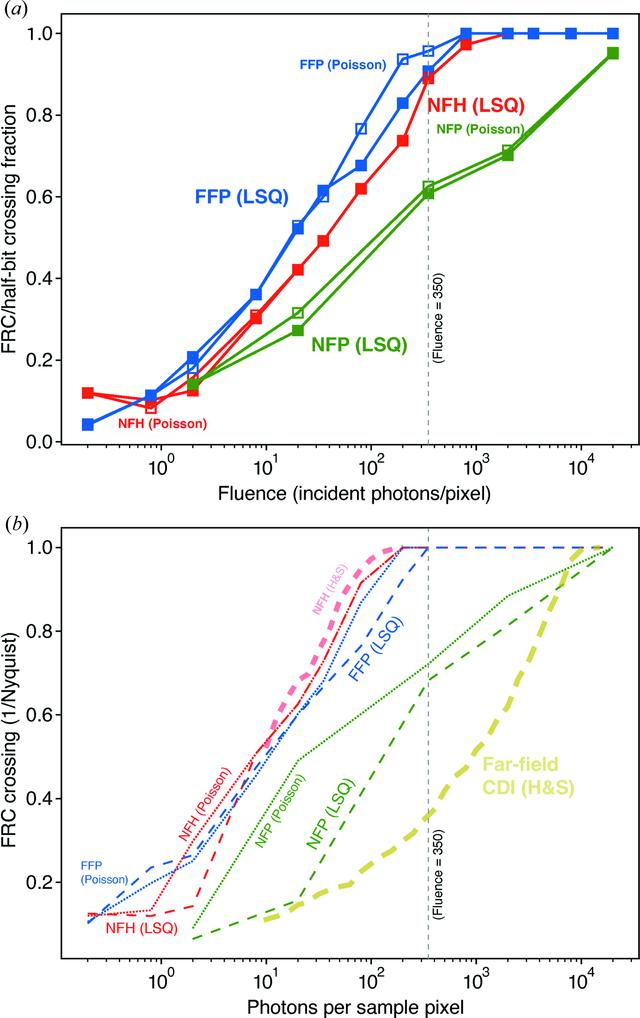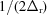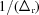disable zoom     view article Figure 6 Values for the crossing between the FRC curves of Fig. 5and the half-bit resolution criterion (van Heel & Schatz, 2005), shown as a fraction of the Nyquist spatial frequency limit of. This is done for NFH, FFP and NFP. In (a), the crossing values are shown for the FRC analysis between reconstructed images obtained from two instances of Poisson noise, as normally required. The curves are not entirely smooth due to the sensitivity of the FRC crossing to the exact noise instance of the FRC curves shown in Fig. 5, but they show that one achieves full spatial resolution with NFH and FFP at fluences near the value of 350 quanta per pixel (shown with a vertical dashed line) estimated after equation (12). In prior work (Hagemann & Salditt, 2017), the FRC crossing analysis was done by comparison of one noise instance with the ground-truth' object of the cell phantom, so (b) shows our results for an equivalent ground-truth' FRC crossing as dashed lines. Also shown in (b) are the approximate results of the previous study (Hagemann & Salditt, 2017) of NFH, labeled with (H&S)' as obtained by tracing of the published figure. [The previous study plotted the FRC crossing as a function of, so we have multiplied the FRC crossing fractions by a factor of 2.] As can be seen, our ground-truth analysis' results and the H&S' results are reasonably consistent for the case of NFH. The previous study also considered far-field CDI [Far-field CDI (H&S)'], where the entire object array is illuminated and a finite-support constraint is applied during iterative reconstruction.JOURNAL OFAPPLIEDCRYSTALLOGRAPHY
ISSN: 1600-5767
Volume 53| Part 3| June 2020| Pages 748-759